## *HYPERFOAM

Keyword type: model definition, material

This option is used to define a hyperfoam material. There is one optional parameters, N. N determines the order of the strain energy potential. Default is N=1. All constants may be temperature dependent.

The hyperfoam strain energy potential takes the form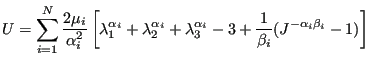(641)

where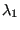,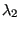and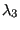are the principal stretches. The parameters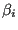are related to the Poisson coefficients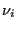by: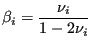(642)

and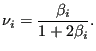(643)

First line:

• *HYPERFOAM
• Enter parameters and their values, if needed

Following line for N=1:

•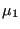.
•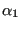.
•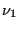.
• Temperature.
Repeat this line if needed to define complete temperature dependence.

Following line for N=2:

•.
•.
•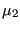.
•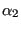.
•.
•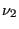.
• Temperature.
Repeat this line if needed to define complete temperature dependence.

Following lines, in a pair, for N=3: First line of pair:

•.
•.
•.
•.
•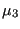.
•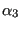.
•.
•.
Second line of pair:
•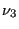.
• Temperature.
Repeat this pair if needed to define complete temperature dependence.

Example:

*HYPERFOAM,N=2
0.164861,8.88413,2.302e-5,-4.81798,0.,0.


defines a hyperfoam material with two terms in the series.

Example files: beamhf.